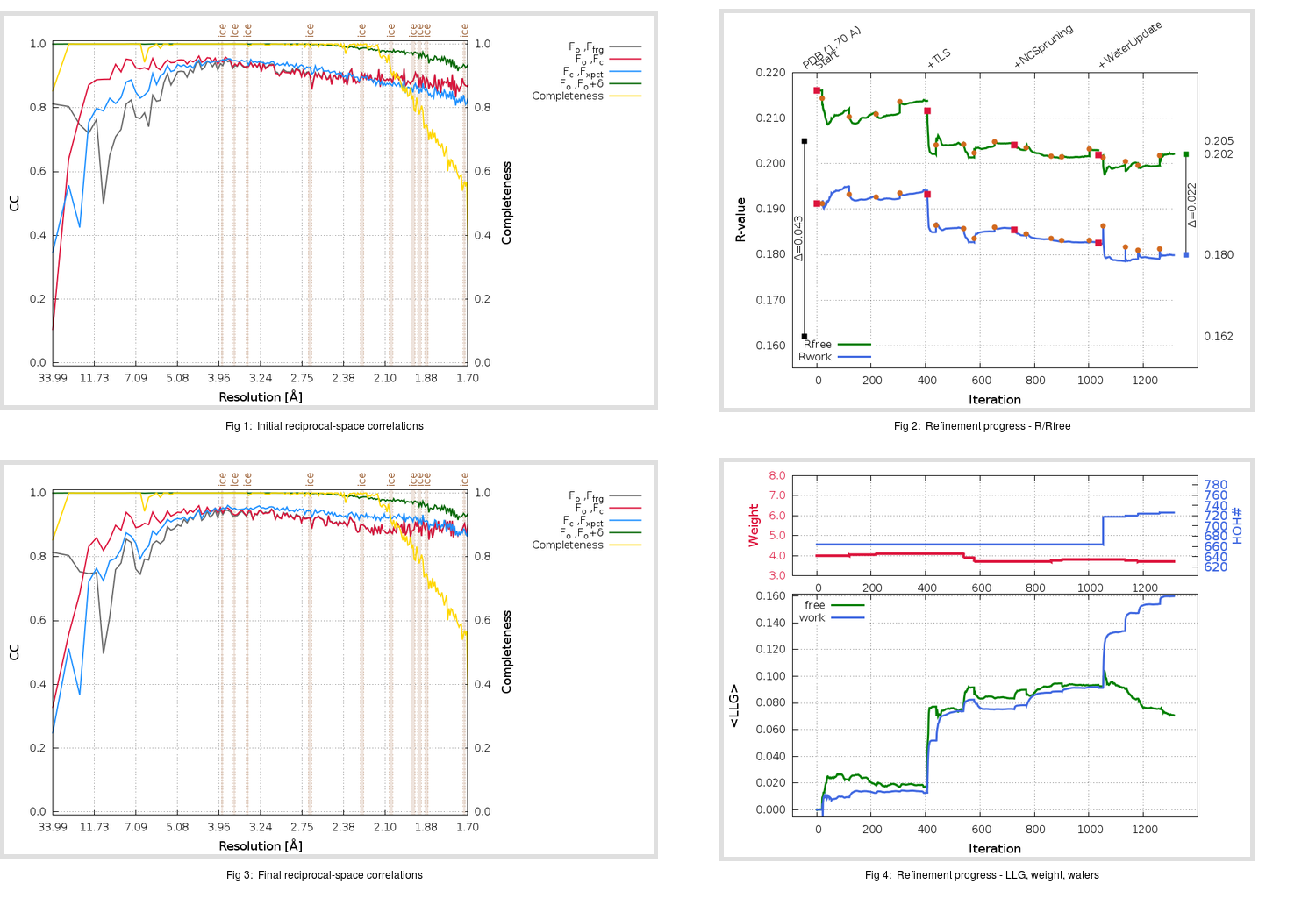Content:

```    Diffraction limits & principal axes of ellipsoid fitted to diffraction cut-off surface:
1.993         1.0000   0.0000   0.0000       a*
1.637         0.0000   1.0000   0.0000       b*
1.634         0.0000   0.0000   1.0000       c*
```

## Deposited

` `
 Date deposited Date data collection Resolution R, Rfree 20200227 20200225 1.70 0.1600 0.2050

Molprobity (CCP4 7.0 version) summary:

```Ramachandran outliers =   0.00 %
favored =  98.17 %
Rotamer outliers      =   0.50 %
C-beta deviations     =     0
Clashscore            =   2.90
RMS(bonds)            =   0.0036
RMS(angles)           =   0.94
MolProbity score      =   1.08
Resolution            =   1.70
R-work                =   0.1600
R-free                =   0.2050
```

```Number of waters      =   664

<B> (all atoms) =   21.21 ( sd =   11.92 ) for       4683 non-hydrogen atoms
<B>   (protein) =   19.30 ( sd =   11.08 ) for       3939 non-hydrogen atoms
<B>     (water) =   31.07 ( sd =   11.31 ) for        664 non-hydrogen atoms
<B>    (others) =   33.32 ( sd =   10.46 ) for         80 non-hydrogen atoms

B min/max       (all non-hydrogen atoms) =    5.20 /   76.18
B min/max   (protein non-hydrogen atoms) =    5.20 /   70.74
B min/max     (water non-hydrogen atoms) =    7.80 /   76.18
B min/max     (other non-hydrogen atoms) =   11.14 /   58.64
```

## BUSTER (re-)refinement

` `

Molprobity (CCP4 7.0 version) summary:

```Ramachandran outliers =   0.00 %
favored =  98.57 %
Rotamer outliers      =   0.50 %
C-beta deviations     =     0
Clashscore            =   1.64
RMS(bonds)            =   0.0123
RMS(angles)           =   1.54
MolProbity score      =   0.91
Resolution            =   1.70
R-work                =   0.1799
R-free                =   0.2021
```

```Number of waters      =   726

<B> (all atoms) =   22.56 ( sd =   12.70 ) for       4745 non-hydrogen atoms
<B>   (protein) =   20.22 ( sd =   11.34 ) for       3939 non-hydrogen atoms
<B>     (water) =   33.64 ( sd =   12.99 ) for        726 non-hydrogen atoms
<B>    (others) =   37.32 ( sd =   12.90 ) for         80 non-hydrogen atoms

B min/max       (all non-hydrogen atoms) =    5.27 /  102.28
B min/max   (protein non-hydrogen atoms) =    5.27 /   74.20
B min/max     (water non-hydrogen atoms) =    7.20 /  102.28
B min/max     (other non-hydrogen atoms) =   11.97 /   80.00
```

Refinement progression:Results:

` `
 File Remark 6VYO_aB_refine.01_04_refine.pdb.gz exact refinement commands are in header 6VYO_aB_refine.01_04_refine.mtz.gz including original deposited data and several re-refinement map coefficients 6VYO_aB_refine.01_04_BUSTER_model.cif.gz including any non-standard compound restraints 6VYO_aB_refine.01_04_BUSTER_refln.cif.gz